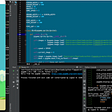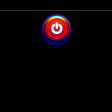# Introduction

Plots in Matplotlib have a hierarchical structure that nests Python objects to create a tree-like structure. Each plot is encapsulated in a Figure object. This Figure is the top-level container of the visualization. It can have multiple axes, which are basically individual plots inside this top-level container.

Furthermore, we again find Python objects that control axes, tick marks, legends, titles, text boxes, the grid, and many other objects. All of these objects can be customized.

The two main components of a plot are as follows:

• Figure: The Figure is an outermost container that allows you to draw multiple plots within it. It not only holds the Axes object but also has the ability to configure the Title.
• Axes: The axes are an actual plot, or subplot, depending on whether you want to plot single or multiple visualizations. Its sub-objects include the x-axis, y-axis, spines, and legends.

Observing this design, we can see that this hierarchical structure allows us to create a complex and customizable visualization.

When looking at the “anatomy” of a Figure (shown in the following diagram), we get an idea about the complexity of a visualization. Matplotlib gives us the ability not only to display data, but also design the whole Figure around it by adjusting the Grid, X and Y ticks, tick labels, and the Legend. This implies that we can modify every single bit of a plot, starting from the Title and Legend, right down to the major and minor ticks on the spines:

Taking a deeper look into the anatomy of a Figure object, we can observe the following components:

• Spines: Lines connecting the axis tick marks
• Title: Text label of the whole Figure object
• Legend: Describes the content of the plot
• Grid: Vertical and horizontal lines used as an extension of the tick marks
• X/Y axis label: Text labels for the X and Y axes below the spines
• Minor tick: Small value indicators between the major tick marks
• Minor tick label: Text label that will be displayed at the minor ticks
• Major tick: Major value indicators on the spines
• Major tick label: Text label that will be displayed at the major ticks
• Line: Plotting type that connects data points with a line
• Markers: Plotting type that plots every data point with a defined marker

In this course, we will focus on Matplotlib’s sub-module, pyplot, which provides MATLAB-like plotting.

# Pyplot Basics

pyplot contains a simpler interface for creating visualizations that allow the users to plot the data without explicitly configuring the Figure and Axes themselves. They are automatically configured to achieve the desired output. It is handy to use the alias plt to reference the imported sub-module, as follows:

`import matplotlib.pyplot as plt`

The following sections describe some of the common operations that are performed when using pyplot.

## Creating Figures

You can use plt.figure() to create a new Figure. This function returns a Figure instance, but it is also passed to the back-end. Every Figure-related command that follows is applied to the current Figure and does not need to know the Figure instance.

By default, the Figure has a width of 6.4 inches and a height of 4.8 inches with a dpi (dots per inch) of 100. To change the default values of the Figure, we can use the parameters fig-size and dpi.

The following code snippet shows how we can manipulate a Figure:

`plt.figure(figsize=(10, 5)) #To change the width and the heightplt.figure(dpi=300) #To change the dpi`

Even though it is not necessary to explicitly create a Figure, this is a good practice if you want to create multiple Figures at the same time.

## Closing Figures

Figures that are no longer used should be closed by explicitly calling plt.close(), which also cleans up memory efficiently.

If nothing is specified, the plt.close() command will close the current Figure. To close a specific Figure, you can either provide a reference to a Figure instance or provide the Figure number. To find the number of a Figure object, we can make use of the number attribute, as follows:

`plt.gcf().number`

The plt.close(‘all’) command is used to close all active Figures. The following example shows how a Figure can be created and closed:

`plt.figure(num=10) #Create Figure with Figure number 10plt.close(10) #Close Figure with Figure number 10`

For a small Python script that only creates a visualization, explicitly closing a Figure isn’t required, since the memory will be cleaned in any case once the program terminates. But if you create lots of Figures, it might make sense to close Figures in between so as to save memory.

## Format Strings

Before we actually plot something, let’s quickly discuss format strings. They are a neat way to specify colors, marker types, and line styles. A format string is specified as [color][marker][line], where each item is optional. If the color argument is the only argument of the format string, you can use matplotlib.colors. Matplotlib recognizes the following formats, among others:

• RGB or RGBA float tuples (for example, (0.2, 0.4, 0.3) or (0.2, 0.4, 0.3, 0.5))
• RGB or RGBA hex strings (for example, ‘#0F0F0F’ or ‘#0F0F0F0F’)

To conclude, format strings are a handy way to quickly customize colors, marker types, and line styles. It is also possible to use arguments, such as color, marker, and linestyle.

## Plotting

With plt.plot([x], y, [fmt]), you can plot data points as lines and/or markers. The function returns a list of Line2D objects representing the plotted data. By default, if you do not provide a format string (fmt), the data points will be connected with straight, solid lines. plt.plot([0, 1, 2, 3], [2, 4, 6, 8]) produces a plot, as shown in the following diagram. Since x is optional and the default values are [0, …, N-1], plt.plot([2, 4, 6, 8]) results in the same plot:

If you want to plot markers instead of lines, you can just specify a format string with any marker type. For example, plt.plot([0, 1, 2, 3], [2, 4, 6, 8], ‘o’) displays data points as circles, as shown in the following diagram:

To plot multiple data pairs, the syntax plt.plot([x], y, [fmt], [x], y2, [fmt2], …) can be used. plt.plot([2, 4, 6, 8], ‘o’, [1, 5, 9, 13], ‘s’) results in the following diagram. Similarly, you can use plt.plot multiple times, since we are working on the same Figure and Axes:

Any Line2D properties can be used instead of format strings to further customize the plot. For example, the following code snippet shows how we can additionally specify the linewidth and markersize arguments:

`plt.plot([2, 4, 6, 8], color='blue', marker='o', linestyle='dashed', linewidth=2, markersize=12)`

Besides providing data using lists or NumPy arrays, it might be handy to use pandas DataFrames, as explained in the next section.

## Plotting Using pandas DataFrames

It is pretty straightforward to use pandas.DataFrame as a data source. Instead of providing x and y values, you can provide the pandas.DataFrame in the data parameter and give keys for x and y, as follows:

`plt.plot('x_key', 'y_key', data=df)`

If your data is already a pandas DataFrame, this is the preferred way.

## Ticks

Tick locations and labels can be set manually if Matplotlib’s default isn’t sufficient. Considering the previous plot, it might be preferable to only have ticks at multiples of ones at the x-axis. One way to accomplish this is to use plt.xticks() and plt.yticks() to either get or set the ticks manually.

plt.xticks(ticks, [labels], [**kwargs]) sets the current tick locations and labels of the x-axis.

Parameters:

• ticks: List of tick locations; if an empty list is passed, ticks will be disabled.
• labels (optional): You can optionally pass a list of labels for the specified locations.
• **kwargs (optional): matplotlib.text.Text() properties can be used to customize the appearance of the tick labels. A quite useful property is rotation; this allows you to rotate the tick labels to use space more efficiently.

Example:

Consider the following code to plot a graph with custom ticks:

`plt.figure(figsize=(6, 3))plt.plot([2, 4, 6, 8], 'o', [1, 5, 9, 13], 's')plt.xticks(ticks=np.arange(4))`

It’s also possible to specify tick labels, as follows:

`plt.figure(figsize=(6, 3))plt.plot([2, 4, 6, 8], 'o', [1, 5, 9, 13], 's')plt.xticks(ticks=np.arange(4), labels=['January', 'February', 'March', 'April'], rotation=20)`

This will result in the following plot:

If you want to do even more sophisticated things with ticks, you should look into tick locators and formatters. For example, would remove the major ticks of the x-axis, and ax.xaxis.set_major_formatter(plt.NullFormatter()) would remove the major tick labels, but not the tick locations of the x-axis.

## Displaying and Saving Figures

plt.show() is used to display a Figure or multiple Figures. To display Figures within a Jupyter Notebook, simply set the %matplotlib inline command at the beginning of the code.

If you forget to use plt.show(), the plot won’t show up. We will learn how to save the Figure in the next section.

The plt.savefig(fname) saves the current Figure. There are some useful optional parameters you can specify, such as dpi, format, or transparent. The following code snippet gives an example of how you can save a Figure:

`plt.figure()plt.plot([1, 2, 4, 5], [1, 3, 4, 3], '-o')plt.savefig('lineplot.png', dpi=300, bbox_inches='tight')#bbox_inches='tight' removes the outer white margins`

## Conclusion

So that’s all for this article , we learnt the basics of figure objects, ticks, labels, formatting line and text, passing pandas data frame as data source and saving a figure.

Hope you enjoyed and see you in the next ones.

Until then you can connect with me on my Instagram😍

--

--

--

## More from Abhijeet Srivastav

CEO Techneophyte | Python Developer | ML Engineer | Data Scientist | Flutter Developer | Penetration Tester | Software Engineer at Infosys

Love podcasts or audiobooks? Learn on the go with our new app.

## Most popular programming language from 1965 to now!## NoSQL Databases Overview## Best Python projects to finish in a weekend## Let’s talk DSL with Kotlin## CSS Tutorial: Animated Shiny Red Button## Abhijeet Srivastav

CEO Techneophyte | Python Developer | ML Engineer | Data Scientist | Flutter Developer | Penetration Tester | Software Engineer at Infosys

## Data Visualisation In Seaborn With Awesome Examples## Data Profiling with Python: Described## Gradient Boosted Trees for Regression in Python